# sklearn.gaussian_process.GaussianProcessClassifier¶

class sklearn.gaussian_process.GaussianProcessClassifier(kernel=None, *, optimizer='fmin_l_bfgs_b', n_restarts_optimizer=0, max_iter_predict=100, warm_start=False, copy_X_train=True, random_state=None, multi_class='one_vs_rest', n_jobs=None)[source]

Gaussian process classification (GPC) based on Laplace approximation.

The implementation is based on Algorithm 3.1, 3.2, and 5.1 from [RW2006].

Internally, the Laplace approximation is used for approximating the non-Gaussian posterior by a Gaussian.

Currently, the implementation is restricted to using the logistic link function. For multi-class classification, several binary one-versus rest classifiers are fitted. Note that this class thus does not implement a true multi-class Laplace approximation.

Read more in the User Guide.

New in version 0.18.

Parameters:
kernelkernel instance, default=None

The kernel specifying the covariance function of the GP. If None is passed, the kernel “1.0 * RBF(1.0)” is used as default. Note that the kernel’s hyperparameters are optimized during fitting. Also kernel cannot be a CompoundKernel.

optimizer‘fmin_l_bfgs_b’, callable or None, default=’fmin_l_bfgs_b’

Can either be one of the internally supported optimizers for optimizing the kernel’s parameters, specified by a string, or an externally defined optimizer passed as a callable. If a callable is passed, it must have the signature:

def optimizer(obj_func, initial_theta, bounds):
# * 'obj_func' is the objective function to be maximized, which
#   takes the hyperparameters theta as parameter and an
#   optional flag eval_gradient, which determines if the
# * 'initial_theta': the initial value for theta, which can be
#   used by local optimizers
# * 'bounds': the bounds on the values of theta
....
# Returned are the best found hyperparameters theta and
# the corresponding value of the target function.
return theta_opt, func_min


Per default, the ‘L-BFGS-B’ algorithm from scipy.optimize.minimize is used. If None is passed, the kernel’s parameters are kept fixed. Available internal optimizers are:

'fmin_l_bfgs_b'

n_restarts_optimizerint, default=0

The number of restarts of the optimizer for finding the kernel’s parameters which maximize the log-marginal likelihood. The first run of the optimizer is performed from the kernel’s initial parameters, the remaining ones (if any) from thetas sampled log-uniform randomly from the space of allowed theta-values. If greater than 0, all bounds must be finite. Note that n_restarts_optimizer=0 implies that one run is performed.

max_iter_predictint, default=100

The maximum number of iterations in Newton’s method for approximating the posterior during predict. Smaller values will reduce computation time at the cost of worse results.

warm_startbool, default=False

If warm-starts are enabled, the solution of the last Newton iteration on the Laplace approximation of the posterior mode is used as initialization for the next call of _posterior_mode(). This can speed up convergence when _posterior_mode is called several times on similar problems as in hyperparameter optimization. See the Glossary.

copy_X_trainbool, default=True

If True, a persistent copy of the training data is stored in the object. Otherwise, just a reference to the training data is stored, which might cause predictions to change if the data is modified externally.

random_stateint, RandomState instance or None, default=None

Determines random number generation used to initialize the centers. Pass an int for reproducible results across multiple function calls. See Glossary.

multi_class{‘one_vs_rest’, ‘one_vs_one’}, default=’one_vs_rest’

Specifies how multi-class classification problems are handled. Supported are ‘one_vs_rest’ and ‘one_vs_one’. In ‘one_vs_rest’, one binary Gaussian process classifier is fitted for each class, which is trained to separate this class from the rest. In ‘one_vs_one’, one binary Gaussian process classifier is fitted for each pair of classes, which is trained to separate these two classes. The predictions of these binary predictors are combined into multi-class predictions. Note that ‘one_vs_one’ does not support predicting probability estimates.

n_jobsint, default=None

The number of jobs to use for the computation: the specified multiclass problems are computed in parallel. None means 1 unless in a joblib.parallel_backend context. -1 means using all processors. See Glossary for more details.

Attributes:
base_estimator_Estimator instance

The estimator instance that defines the likelihood function using the observed data.

kernel_kernel instance

Return the kernel of the base estimator.

log_marginal_likelihood_value_float

The log-marginal-likelihood of self.kernel_.theta

classes_array-like of shape (n_classes,)

Unique class labels.

n_classes_int

The number of classes in the training data

n_features_in_int

Number of features seen during fit.

New in version 0.24.

feature_names_in_ndarray of shape (n_features_in_,)

Names of features seen during fit. Defined only when X has feature names that are all strings.

New in version 1.0.

GaussianProcessRegressor

Gaussian process regression (GPR).

References

Examples

>>> from sklearn.datasets import load_iris
>>> from sklearn.gaussian_process import GaussianProcessClassifier
>>> from sklearn.gaussian_process.kernels import RBF
>>> kernel = 1.0 * RBF(1.0)
>>> gpc = GaussianProcessClassifier(kernel=kernel,
...         random_state=0).fit(X, y)
>>> gpc.score(X, y)
0.9866...
>>> gpc.predict_proba(X[:2,:])
array([[0.83548752, 0.03228706, 0.13222543],
[0.79064206, 0.06525643, 0.14410151]])


Methods

 fit(X, y) Fit Gaussian process classification model. get_params([deep]) Get parameters for this estimator. log_marginal_likelihood([theta, ...]) Return log-marginal likelihood of theta for training data. Perform classification on an array of test vectors X. Return probability estimates for the test vector X. score(X, y[, sample_weight]) Return the mean accuracy on the given test data and labels. set_params(**params) Set the parameters of this estimator.
fit(X, y)[source]

Fit Gaussian process classification model.

Parameters:
Xarray-like of shape (n_samples, n_features) or list of object

Feature vectors or other representations of training data.

yarray-like of shape (n_samples,)

Target values, must be binary.

Returns:
selfobject

Returns an instance of self.

get_params(deep=True)[source]

Get parameters for this estimator.

Parameters:
deepbool, default=True

If True, will return the parameters for this estimator and contained subobjects that are estimators.

Returns:
paramsdict

Parameter names mapped to their values.

property kernel_

Return the kernel of the base estimator.

Return log-marginal likelihood of theta for training data.

In the case of multi-class classification, the mean log-marginal likelihood of the one-versus-rest classifiers are returned.

Parameters:
thetaarray-like of shape (n_kernel_params,), default=None

Kernel hyperparameters for which the log-marginal likelihood is evaluated. In the case of multi-class classification, theta may be the hyperparameters of the compound kernel or of an individual kernel. In the latter case, all individual kernel get assigned the same theta values. If None, the precomputed log_marginal_likelihood of self.kernel_.theta is returned.

If True, the gradient of the log-marginal likelihood with respect to the kernel hyperparameters at position theta is returned additionally. Note that gradient computation is not supported for non-binary classification. If True, theta must not be None.

clone_kernelbool, default=True

If True, the kernel attribute is copied. If False, the kernel attribute is modified, but may result in a performance improvement.

Returns:
log_likelihoodfloat

Log-marginal likelihood of theta for training data.

Gradient of the log-marginal likelihood with respect to the kernel hyperparameters at position theta. Only returned when eval_gradient is True.

predict(X)[source]

Perform classification on an array of test vectors X.

Parameters:
Xarray-like of shape (n_samples, n_features) or list of object

Query points where the GP is evaluated for classification.

Returns:
Cndarray of shape (n_samples,)

Predicted target values for X, values are from classes_.

predict_proba(X)[source]

Return probability estimates for the test vector X.

Parameters:
Xarray-like of shape (n_samples, n_features) or list of object

Query points where the GP is evaluated for classification.

Returns:
Carray-like of shape (n_samples, n_classes)

Returns the probability of the samples for each class in the model. The columns correspond to the classes in sorted order, as they appear in the attribute classes_.

score(X, y, sample_weight=None)[source]

Return the mean accuracy on the given test data and labels.

In multi-label classification, this is the subset accuracy which is a harsh metric since you require for each sample that each label set be correctly predicted.

Parameters:
Xarray-like of shape (n_samples, n_features)

Test samples.

yarray-like of shape (n_samples,) or (n_samples, n_outputs)

True labels for X.

sample_weightarray-like of shape (n_samples,), default=None

Sample weights.

Returns:
scorefloat

Mean accuracy of self.predict(X) w.r.t. y.

set_params(**params)[source]

Set the parameters of this estimator.

The method works on simple estimators as well as on nested objects (such as Pipeline). The latter have parameters of the form <component>__<parameter> so that it’s possible to update each component of a nested object.

Parameters:
**paramsdict

Estimator parameters.

Returns:
selfestimator instance

Estimator instance.

## Examples using sklearn.gaussian_process.GaussianProcessClassifier¶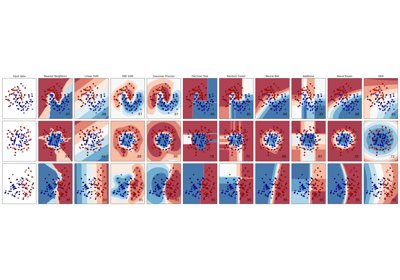Classifier comparison

Classifier comparison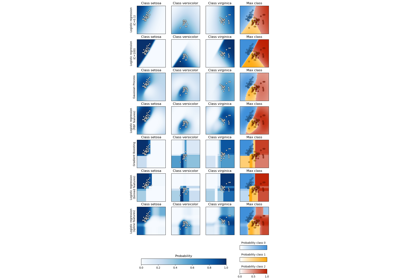Plot classification probability

Plot classification probability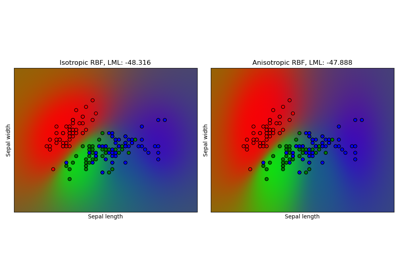Gaussian process classification (GPC) on iris dataset

Gaussian process classification (GPC) on iris dataset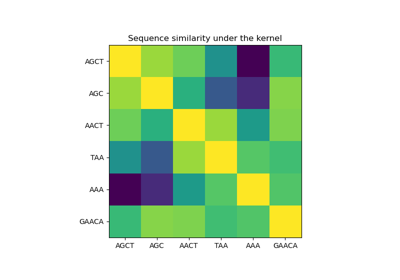Gaussian processes on discrete data structures

Gaussian processes on discrete data structures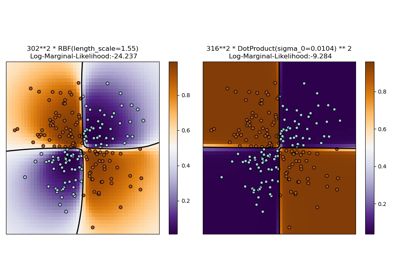Illustration of Gaussian process classification (GPC) on the XOR dataset

Illustration of Gaussian process classification (GPC) on the XOR dataset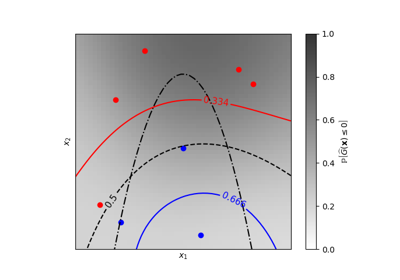Iso-probability lines for Gaussian Processes classification (GPC)

Iso-probability lines for Gaussian Processes classification (GPC)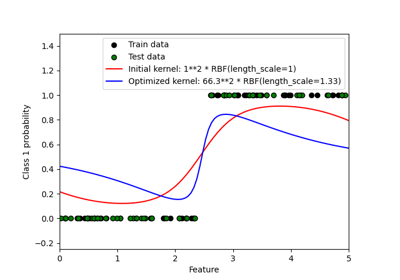Probabilistic predictions with Gaussian process classification (GPC)

Probabilistic predictions with Gaussian process classification (GPC)